Disclaimer: This is an example of a student written essay.
Click here for sample essays written by our professional writers.

Any scientific information contained within this essay should not be treated as fact, this content is to be used for educational purposes only and may contain factual inaccuracies or be out of date.

# Electric Drive System Design, Simulation and Construction

 ✅ Paper Type: Free Essay ✅ Subject: Engineering ✅ Wordcount: 2702 words ✅ Published: 31st Aug 2017

Abstract

In this experiment, three phase flexible inverter was used to drive a Brush Less Direct Current (BLDC) machine. Three experiments steps use three-phase inverter which is connected to resistor and inductor load and BLDC machine to examine and measure 1) Pulse Mode Modulation (PMW); 2) Three wave signal sinusoidal and 3) BLDC signal sequence. Computer simulation using MATLAB Simulink is used to analyze the laboratory result and software calculation. The speed and torque of BLDC machine can be controlled by controller using PWM technique. This BLDC machine can be modelled as Resistor and Inductor Load.

Get Help With Your Essay

If you need assistance with writing your essay, our professional essay writing service is here to help!

## 1.1 Background

Many electrical load (e.g. electric motor, lighting) need a wide of range voltage, current, frequency and phase angle which is converted from electrical source (e.g. electric grid, battery) with constant voltage, frequencies, current and phase angles. This conversion mechanism use power electronics converter and one application of converter is inverter which convert DC input into AC output . In this experiment, this inverter will provide a variety of voltage and frequency to drive BLDC machine in order to operate at a specific speed and torque.

## 1.2 Module Aims

The aim of this experiment is to understand design, construction, simulation, and testing of an electrical drive system through practical experience. The procedure of this project can be divide into following steps.

• Research electrical drive technology.
• Construction of an electric drive.
• Simulate and understand an electric drive system.
• Experimental test, analysis and verification of the system
• Diagrammatic interpretation of software operation
• Production of a written technical report.

## 2.1 Pulse Width Modulation

In this experiment, the circuit use 3 pairs of MOSFETs as shown in figure 2.1. Having set the PIC software to the PWM test mode for the controller, measurements was taken on terminal point in three pairs (J1-J2, J3-J4 and J5-J6) which indicate gate-on voltage.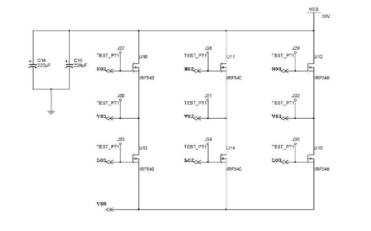Figure 2.1. 3-phase MOSFET arrangement diagram.

The gate-on voltage on terminal J1-J2 over time can be shown in figure xx, it consists of time-on (T-on) and time-off (T-off). Time switching period can be calculated as T=t-on+t-off and duty cycle as ratio of time-on (T-on) over time switching period. The 3 pairs of MOSFET have relatively the same switching frequency and gate-on voltage, but in the different percentage of duty cycle, as complete result shown in table xxx. From figure xx, dead time is calculated as time span from J1 when voltage start to off and J2 when voltage start to on.

PMW switching frequency =

Duty cycle =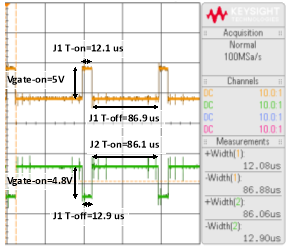Figure 2.2. PWM signal for J1 (Yellow) and J2 (Green): time-on, time-off and voltage on.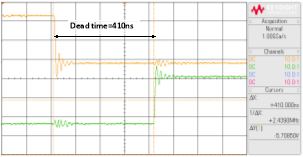Figure 2.3.  PWM signal: dead time between J1 (Yellow) and J2 (Green).

Table 2.1. PWM Signal (J1 to J6)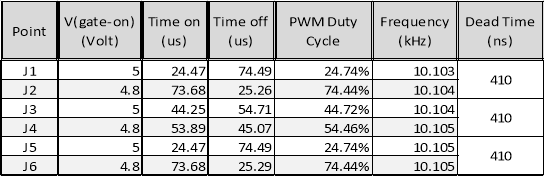## 2.2 Three Phase Sine Wave Generator

In this experiment, the controller is programmed with test 2 test to convert from DC supply to 3-phase AC output. The output of inverter connected to electrical load (resistor and inductor) and then voltage is measured between resistor R1. The frequency of AC output can be altered by the R22 potentiometer.

By varying the setting of potentiometer, the result between resistor R1 (V1-V2) can be shown in figure 2.1 for minimum, figure 2.2 for middle, and figure 2.3 for maximum setting of potentiometer.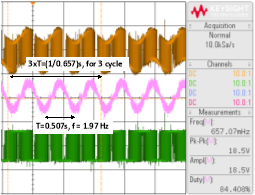Figure 2.4. Inverter frequency (f=1.97Hz) in the minimum setting of potentiometer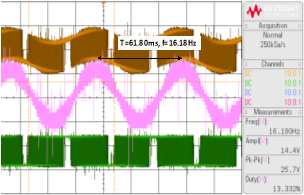Figure 2.5.  Inverter frequency (f=16.18Hz) in the middle setting of potentiometer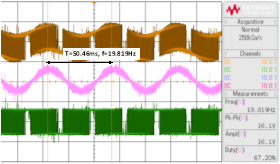Figure 2.6. Inverter frequency (f=19.82Hz) in the maximum setting of potentiometer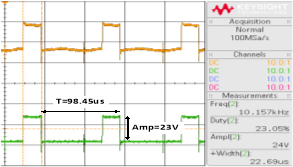Figure 2.7. PWM signal (f=10.16kHz) while at the duty cycle 23%.

## 2.3   BLDC Motor Control

In the test-3, the controller is programmed with test 3 test to convert from DC supply to operate and control a three phase BLDC machine. BLDC machine is equipped with three Hall Effect sensors, their function is to sense the rotor position to the controller in order to make MOSFETs can make certain switching arrangement to produce a particular speed for BLDC motor.

After inverter connected to hall effect sensor and to power supply of BLDC motor, the results can be shown for the sequence position between H1-H2 (figure xx), between H1-H3 (figure xx) and also the total sequence position between H1-H2-H3 in figure xx. The supply voltage for BLDC, phase A voltage is can be shown in figure xx and figure, which magnitude at around 25 V but the duty cycle fluctuate between range 37% up to 62%.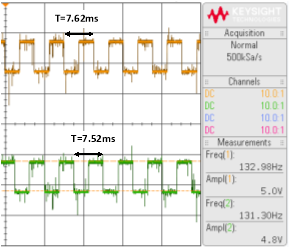Figure 2.8. H1 (Yellow) and H2 (Green) sequence at almost similar frequency (f1=132Hz and f2=131Hz)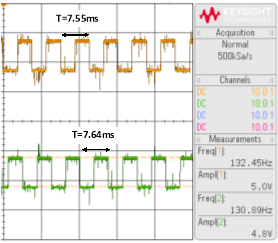Figure 2.9.  H1 (Yellow) and H3 (Green) sequence at almost similar frequency (f1=132Hz and f3=130Hz)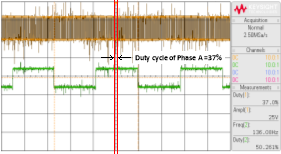Figure 2.10. Relation between H1 (state 1) and voltage phase A (duty 37%).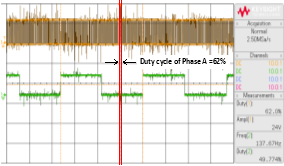Figure 2.11. Relation between H1 (state 0) and voltage phase A (duty 62%).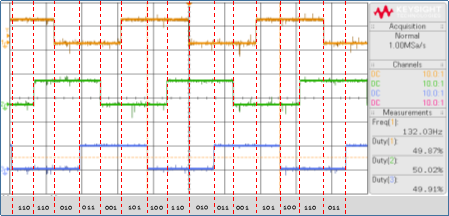Figure 2.12. H1-H2-H3 command sequence.

## 2.4   Variable DC Supply Simulation

In this Matlab simulation, DC supply was connected to resistive-inductive load with the value of R=47Ohm and L=33mH. DC output was produced by compare the triangle waveform and a reference setting point. The triangle waveform magnitude has minimum value -1 and maximum value +1 with a frequency 20kHz. The reference setting point was set at 0, 0.5 and 1 which represent duty cycle (D=0%, D=50% and D=100%).

The shape of current waveform with duty cycle can be shown in figure xx. There are several screens captures with a variation of 1) the reference setting point (figure xx), 2) the triangle switching frequency and 3) the value of inductance.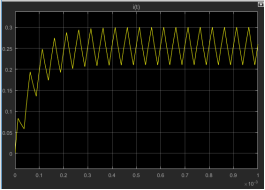Figure 2.13. The output current with duty cycle D=50% and swithing frequency fs=20kHz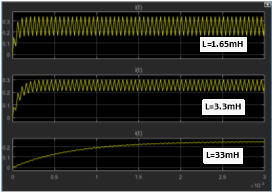Figure 2.14. The output current with different inductance duty cycle (D=0%, D=50%, and D=100%)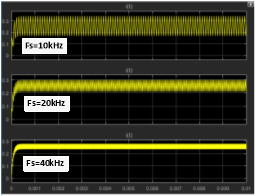Figure 2.15. The output current with different switching frequency (fs=10kHz, fs=20kHz, and fs=40kHz)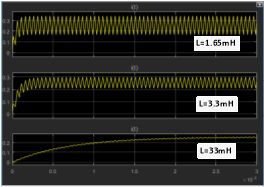Figure 2.16. The output current with different inductance value (L=1.65mH, L=3.3mH, and L=33mH)

## 2.5   Variable AC Supply Simulation

In this AC supply, AC supply was also connected to resistive-inductive load with the value of R=47Ohm and L=33mH. AC output was produced by compare the triangle waveform and a sinusoidal control signal. The triangle waveform magnitude has minimum value -1 and maximum value +1 with a frequency 10kHz. The reference was a sine wave with frequency 50 Hz and magnitude varying from maximum +1 and minimum -1.

The shape of current waveform with duty cycle can be shown in figure xx. There are several screens captures with a variation of 1) the sine wave reference signal (figure xx), 2) the triangle switching frequency and 3) the value of inductance.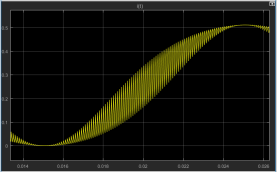Figure 2.17. The output current with a sine wave reference setting fc=50Hz and a switching frequency fs=10kHz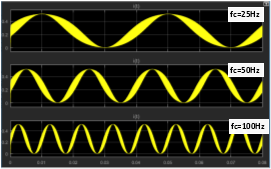Figure 2.18.  The output current with different sine wave reference setting (fc=25Hz, fc=50Hz, and fc=100Hz)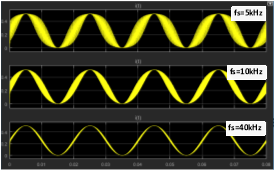Figure 2.19. The output current with different switching frequency (fs=10kHz, fs=20kHz, and fs=40kHz)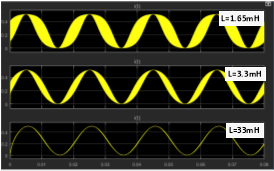Figure 2.20. The output current with different inductance value (L=1.65mH, L=3.3mH, and L=33mH)

## 3.1 Pulse Width Modulation (PWM)

This MS Word template (.dot file) was prepared by Dr. Jonathan I. Maletic in the Department of Computer Science at Kent State University.  This is Version 1.0.  It is a template for Thesis/Dissertations for the College of Arts and Science at KSU.

## 3.2 Three Phase Sine Wave Generator

In this Matlab simulation, DC supply was connected to resistive-inductive load with the value of R=47Ohm and L=33mH. DC output was produced by compare the triangle waveform and a reference setting point. The triangle waveform magnitude has minimum value -1 and maximum value +1 with a frequency 20kHz. The reference setting point was set at 0, 0.5 and 1 which represent duty cycle (D=0%, D=50% and D=100%).

The shape of current waveform with duty cycle can be shown in figure xx. There are several screens captures with a variation of 1) the reference setting point (figure xx), 2) the triangle switching frequency and 3) the value of inductance.

## 3.3 BLDC Motor Control

In this AC supply, AC supply was also connected to resistive-inductive load with the value of R=47Ohm and L=33mH. AC output was produced by compare the triangle waveform and a sinusoidal control signal. The triangle waveform magnitude has minimum value -1 and maximum value +1 with a frequency 10kHz. The reference was a sine wave with frequency 50 Hz and magnitude varying from maximum +1 and minimum -1.

The shape of current waveform with duty cycle can be shown in figure xx. There are several screens captures with a variation of 1) the sine wave reference signal (figure xx), 2) the triangle switching frequency and 3) the value of inductance.

## 3.4 Variable DC Supply Simulation

In this AC supply, AC supply was also connected to resistive-inductive load with the value of R=47Ohm and L=33mH. AC output was produced by compare the triangle waveform and a sinusoidal control signal. The triangle waveform magnitude has minimum value -1 and maximum value +1 with a frequency 10kHz. The reference was a sine wave with frequency 50 Hz and magnitude varying from maximum +1 and minimum -1.

The shape of current waveform with duty cycle can be shown in figure xx. There are several screens captures with a variation of 1) the sine wave reference signal (figure xx), 2) the triangle switching frequency and 3) the value of inductance.

## 3.5 Variable AC Supply Simulation

In this AC supply, AC supply was also connected to resistive-inductive load with the value of R=47Ohm and L=33mH. AC output was produced by compare the triangle waveform and a sinusoidal control signal. The triangle waveform magnitude has minimum value -1 and maximum value +1 with a frequency 10kHz. The reference was a sine wave with frequency 50 Hz and magnitude varying from maximum +1 and minimum -1.

The shape of current waveform with duty cycle can be shown in figure xx. There are several screens captures with a variation of 1) the sine wave reference signal (figure xx), 2) the triangle switching frequency and 3) the value of inductance.

## Cite This Work

To export a reference to this article please select a referencing stye below:

View all

## DMCA / Removal Request

If you are the original writer of this essay and no longer wish to have your work published on UKEssays.com then please:

Related Services

Related Lectures

Study for free with our range of university lectures!Freelance Writing Jobs

Looking for a flexible role?
Do you have a 2:1 degree or higher?

Study Resources

Free resources to assist you with your university studies!